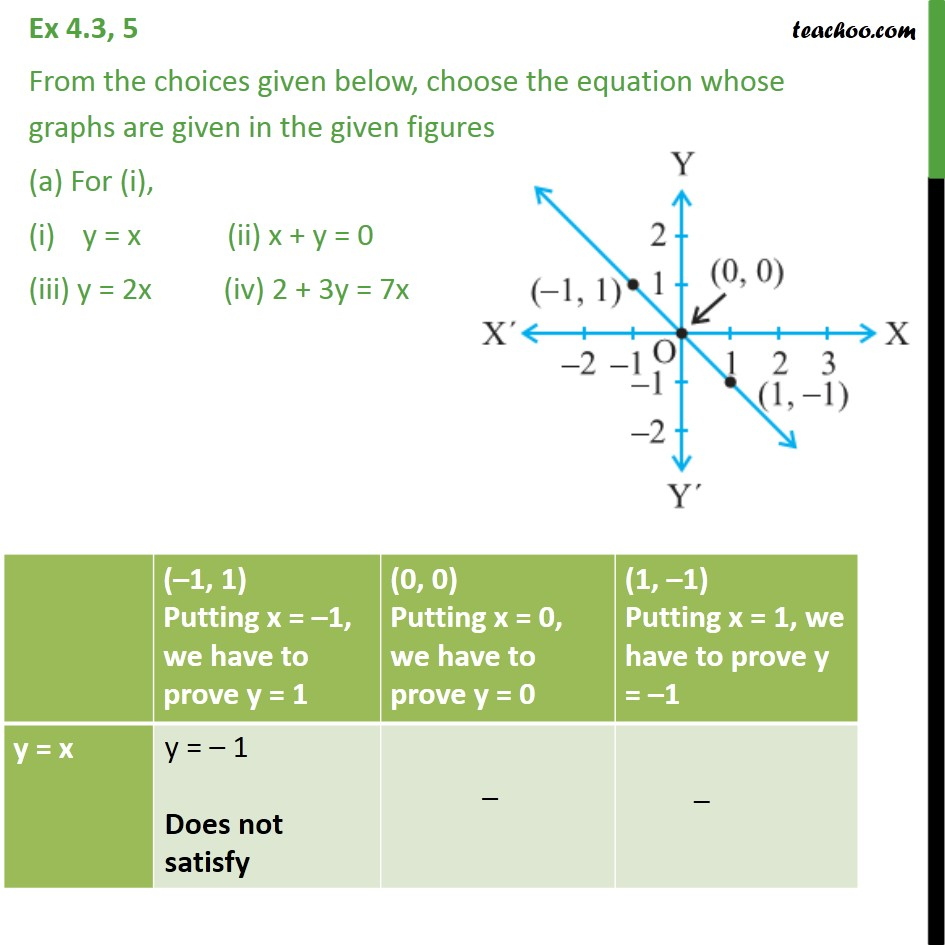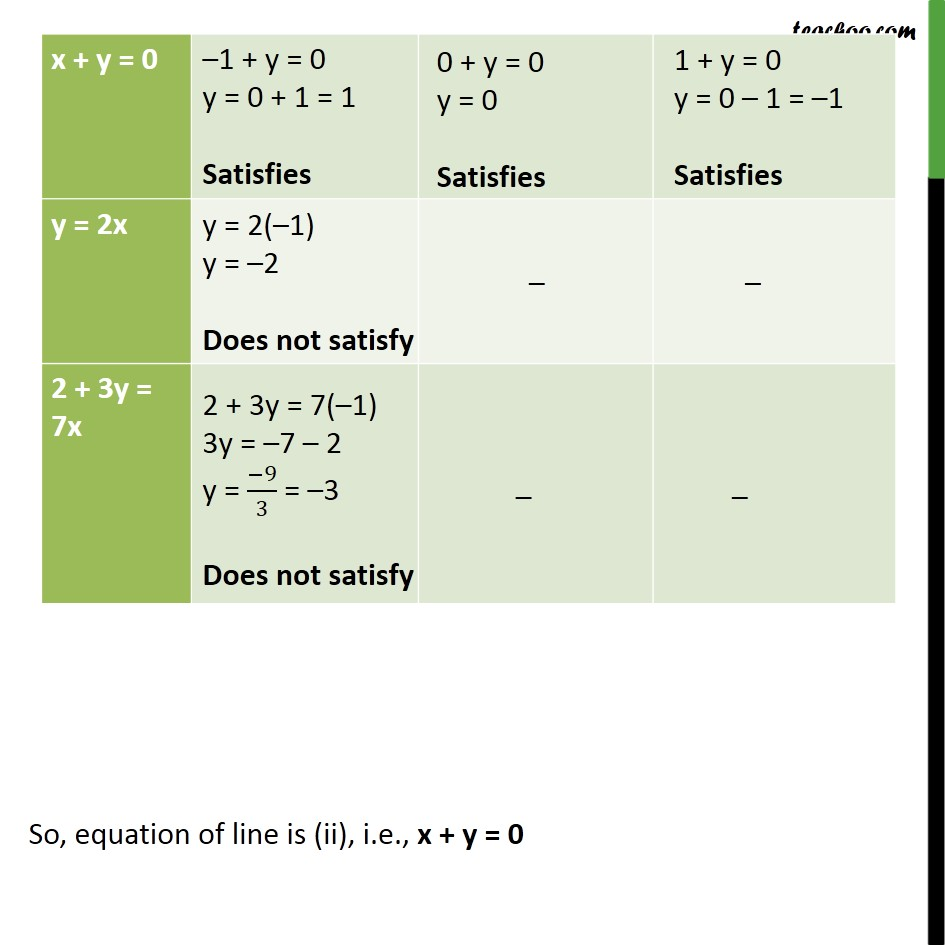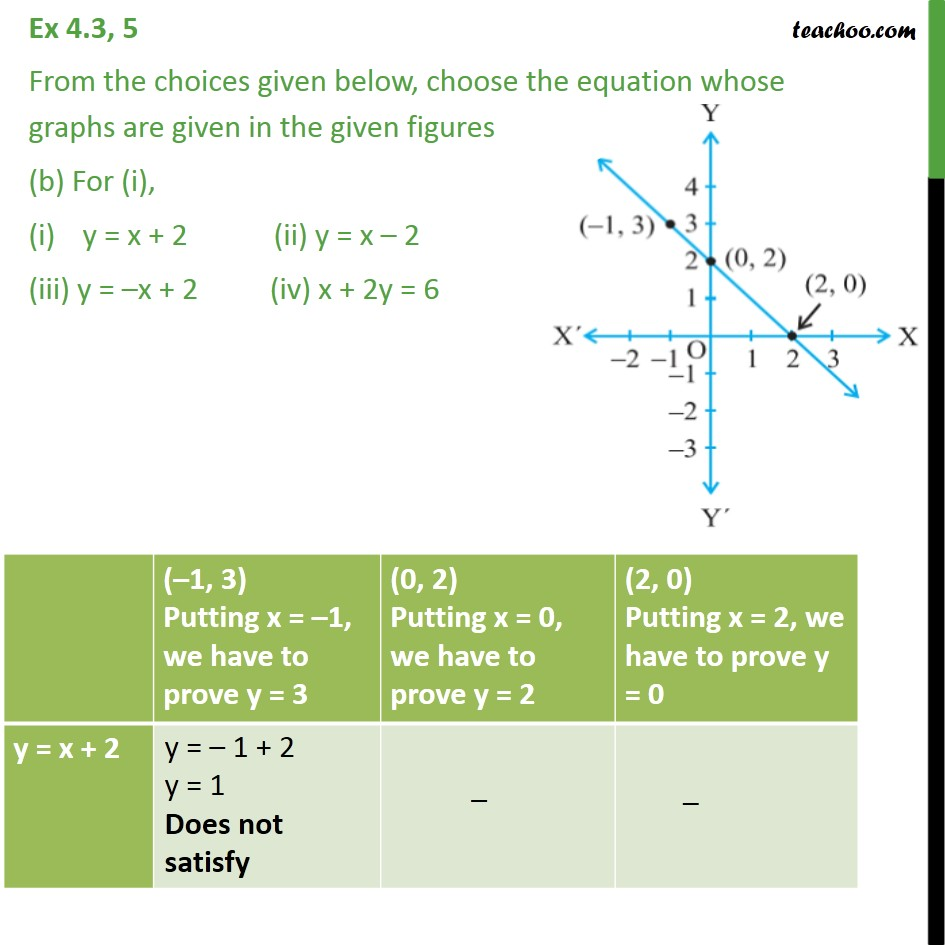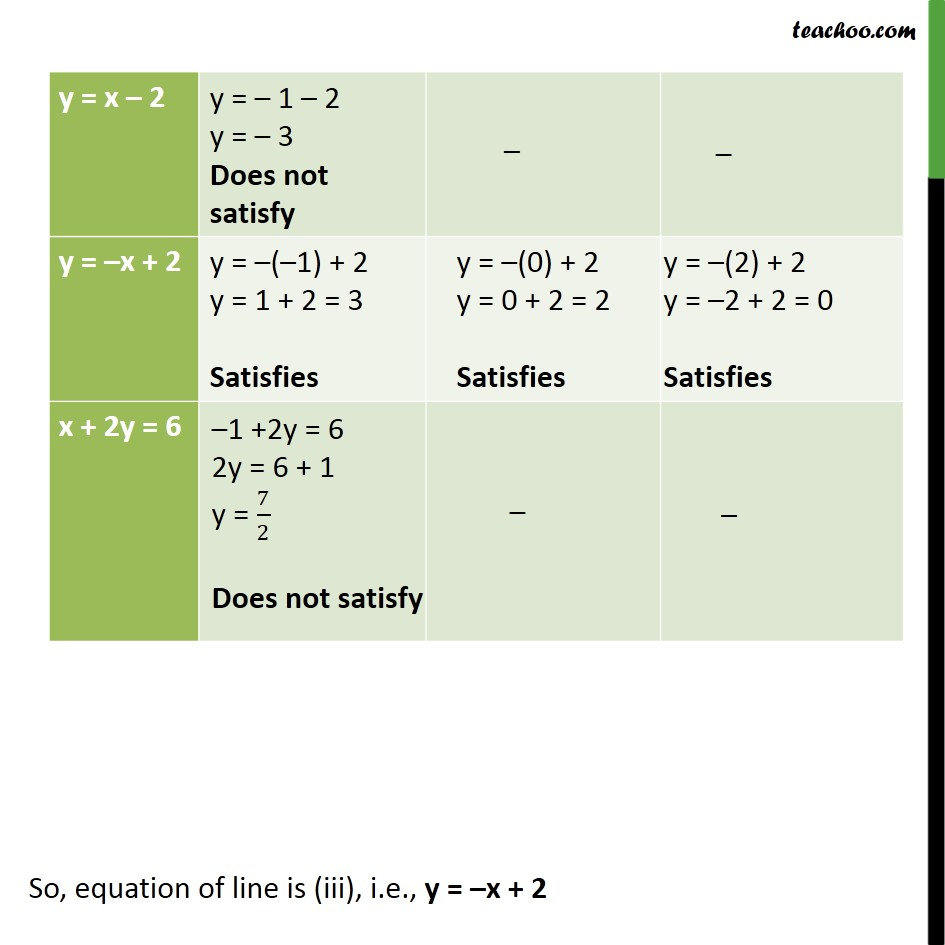Subscribe to our Youtube Channel - https://you.tube/teachoo

1. Chapter 4 Class 9 Linear Equations in Two Variables
2. Concept wise
3. Graph of linear equations

Transcript

Ex 4.3, 5 From the choices given below, choose the equation whose graphs are given in the given figures (a) For (i), y = x (ii) x + y = 0 (iii) y = 2x (iv) 2 + 3y = 7x So, equation of line is (ii), i.e., x + y = 0 Ex 4.3, 5 From the choices given below, choose the equation whose graphs are given in the given figures (b) For (i), y = x + 2 (ii) y = x 2 (iii) y = x + 2 (iv) x + 2y = 6 So, equation of line is (iii), i.e., y = x + 2

Graph of linear equations